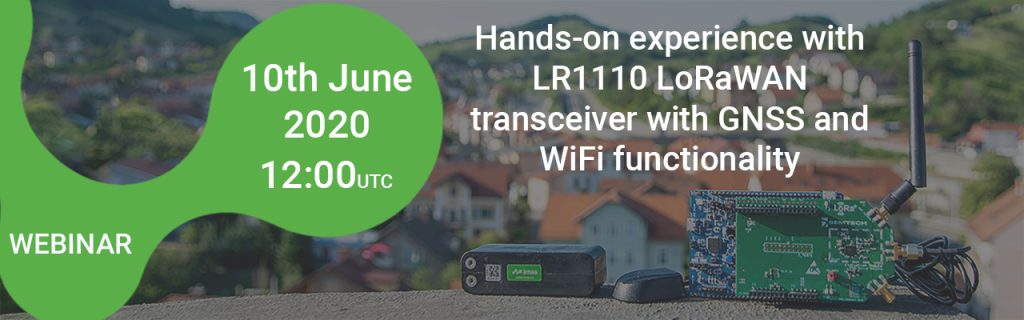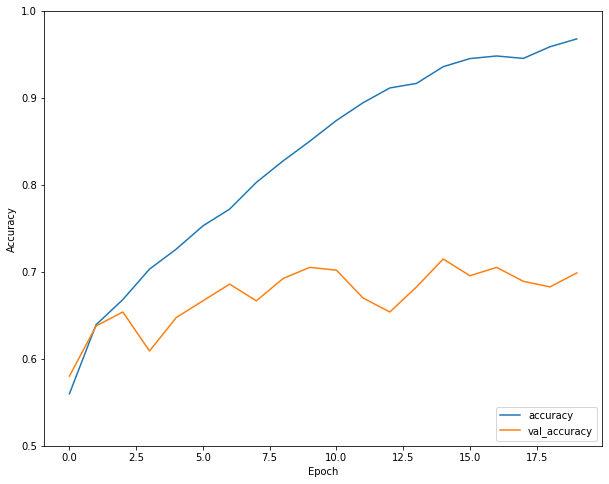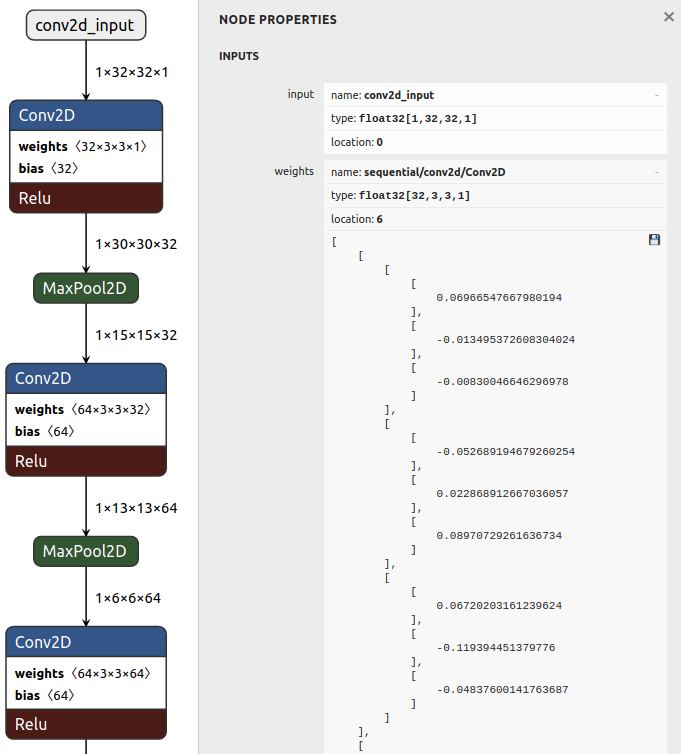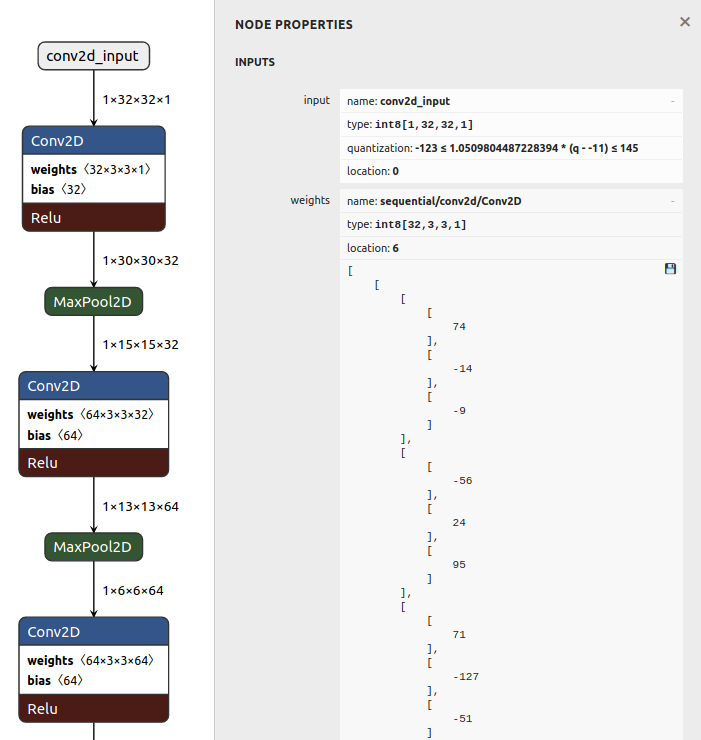# Creating a simple Keras model for inference on microcontrollers – part 1

Author: Marko Sagadin, student intern at IRNAS

In the past few years, there has been slow but constant push to run neural networks on small embedded devices. The most obvious benefit this feature can bring is not needing to send data over the radio to a server for computation. We can do everything we need to do on the device itself, thus saving the energy that would be otherwise used for wireless transmission.

We have already seen examples of products such as Amazon’s Echo or Google’s Google Home that listen all the time for a specific keyword and only send your data to the server after hearing that word. A specific chip was required for such operation in the past, but now, thanks to TensorFlow Lite for Microcontrollers, we can flash a pre-trained neural network model to a common microcontroller and run it on the device.

This article is the first in the series where we will see how to train our model and how to prepare it to run on a microcontroller. The second article will deal with running that model on a microcontroller and comparing the results of inference.

### Overview of what we will do

We will run this demonstration following this Python notebook where we will create a simple Keras model of convolutional neural network that will be able to classify small greyscale images. We will use the well known CIFAR-10 image dataset. After downloading the dataset, we will pre-process the images which will be followed by model training. After training the model, we will convert it to the format that a microcontroller can work with.

To keep things simple, our model will only just be able to recognize 3 different classes (with lots of room for improvement). The purpose of this article is not to be a lesson in CNN architecture or best practises for dealing with image data, but is rather to show the brief workflow from the start to the finish, that can be applied to your specific use case. That comprises starting with a dataset, processing it, creating a model and converting it into something that can be run on a microcontroller.

It is recommended to upload the mentioned notebook to your Google Drive and open it within Google Colaboratory. The colaboratory provides you with Jupyter-like environment where you can run Python notebooks. Numpy, TensorFlow and other required modules are already installed, they just need to be imported. That way you can immediately start running the code!

That is all, let’s get started!### WEBINAR: Hands-on experience with LR1110 LoRaWAN transceiver with GNSS and WiFi functionality

Semtech LR1110 LoRaWAN transceiver with GNSS and WiFi scanning evaluation has shown promising results. To best share our hands-on experience at the first instance we are organizing this session to explore the capabilities of the solution and share our initial experience.

### 1. Mount the Google Drive

First, we need to mount our Google Drive, so we can write and read files to it. We also need to append the location of our folder where we have Python notebook to Python sys path. My location is /gdrive/My Drive/Colab Notebooks/ML_tensorflow_article. Yours will be different, make sure that you append it to the path, do not forget to use the escape character (backslash \ ) before any spaces if your path contains them. After running cell below, you will be prompted to enter authorisation code. You can use cd and ls commands to check if your path is correct.

from Google.colab import drive
import sys

# Mount gdrive
drive.mount('/gdrive')

# Check path, see if python notebook is inside correct folder
%cd /gdrive/My\ Drive/Colab\ Notebooks/ML_tensorflow_article
%ls

# Append location of our folder to python sys path
sys.path.append('/gdrive/My\ Drive/Colab\ Notebooks/ML_tensorflow_article')

### 2. Setup

Import needed modules and make sure that matplotlib graphs are shown inline.

import numpy as np
import matplotlib.pyplot as plt
import tensorflow as tf

%matplotlib inline
plt.rcParams['figure.figsize'] = (10.0, 8.0) # set default size of plots
plt.rcParams['image.interpolation'] = 'nearest'
plt.rcParams['image.cmap'] = 'gray'

%autoreload 2

Just to mention a little about the dataset: CIFAR-10 dataset consists of 60000 32×32 colour images in 10 classes, with 6000 images per class. There are 50000 training images and 10000 test images. Dataset includes pictures of dogs, cats, cars, planes, frogs and others.

# Load CIFAR10 dataset, we can use TensorFlow for this

print("Number of pictures in train dataset: {}".format(train_dataset.shape))
print("Number of pictures in test dataset: {}".format(test_dataset.shape))
print("Picture resolution: {} x {}".format(train_dataset.shape, train_dataset.shape))
print("Number of color channels: {}".format(train_dataset.shape))
print("Shape of train labels: {}".format(train_dataset.shape))


Outputs:

Downloading data from https://www.cs.toronto.edu/~kriz/cifar-10-python.tar.gz
170500096/170498071 [==============================] - 4s 0us/step
Number of pictures in train dataset: 50000
Number of pictures in test dataset: 10000
Picture resolution: 32 x 32
Number of color channels: 3
Shape of train labels: (50000, 1)

### 4. Create validation set

We will set aside 1000 images from the train set to form our validation set. We will use it later in the training. In the process we will also separate labels from pictures, this will enable us easier handling of data.

num_training=49000
num_validation=1000
num_test=1000

# Separate labels from pictures
X_train_whole = train_dataset
y_train_whole = train_dataset
X_test_whole = test_dataset
y_test_whole = test_dataset

mask = list(range(num_training, num_training + num_validation))

y_test = y_test_whole[mask]

### 5. Visualize pictures

Let’s see what kind of pictures are inside CIFAR-10 dataset.

classes = ['plane', 'car', 'bird', 'cat', 'deer', 'dog', 'frog', 'horse', 'ship', 'truck']
num_classes = len(classes)
samples_per_class = 6
for y, cls in enumerate(classes):
idxs = np.flatnonzero(y_train == y)
idxs = np.random.choice(idxs, samples_per_class, replace=False)
for i, idx in enumerate(idxs):
plt_idx = i * num_classes + y + 1
plt.subplot(samples_per_class, num_classes, plt_idx)
plt.imshow(X_train_rgb[idx])
plt.axis('off')
if i == 0:
plt.title(cls)
plt.show()### 6. Convert Color Into Greyscale

We can scale each colour with some factor and add them up to create a greyscale image. In this example, a linear approximation of gamma-compression-corrected version is used, but there are multiple other ways to approach this operation (read more on greyscale conversion here).

The equation that we will use is:
Y’ = 0.299R’ + 0.587G’ + 0.114B’

def rgb2gray(rgb):
rgb[...,0] = rgb[...,0] * 0.2989  # Red channel
rgb[...,1] = rgb[...,1] * 0.5870  # Green channel
rgb[...,2] = rgb[...,2] * 0.1140  # Blue channel

# We want to keep dimensions same so that we go from
# (49000, 32, 32 ,3) to (49000, 32, 32, 1) and not to (49000, 32, 32)
return np.around(np.sum(rgb, axis=3, keepdims=True))

# Convert all data into grayscale and drop decimal part
X_train = rgb2gray(X_train_rgb)
X_val = rgb2gray(X_val_rgb)
X_test = rgb2gray(X_test_rgb)

### 7. Extract 3 classes

Images are now greyscale. Before doing mean normalization we should get out only a few categories that we actually want to train on. Let’s extract 3 classes: cats, dogs and frogs.

# Position of correct classes, cat will be marked as 3, dog as 5, frog as 6
classes = ['plane', 'car', 'bird', 'cat', 'deer', 'dog', 'frog', 'horse', 'ship', 'truck']

# Extract 3 classes
mask = (y_train == 3) | (y_train == 5) | (y_train == 6)

mask = (y_val == 3) | (y_val == 5) | (y_val == 6)

mask = (y_test == 3) | (y_test == 5) | (y_test == 6)

# We adjust labels of the classes to be 0,1,2 and not 3,5,6
'''
We want labels to be 0,1,2 so we will subtract from existing ones
'''
y[y == 3] -= 3
y[y == 5] -= 4
y[y == 6] -= 4

adjust_classes(y_test)

### 8. Preprocessing

Mean subtraction is the most common form of pre-processing. It involves subtracting the mean across every individual feature in the data, and has the geometric interpretation of centering the cloud of data around the origin along every dimension. This prevents gradients to be either positive or negative, which would their values harder to converge. Many algorithms show better performances when the dataset is symmetric (with a zero-mean).

Another preprocessing technique is normalization which squeezes input values between -1 and 1. This is used when input values are widely different which in case of pixels where values range from 0 to 255 (before mean subtraction) is not really necessary.

# Normalize the data: subtract the mean image
mean_image = np.around(np.mean(X_train, axis=0))
X_train = X_train - mean_image
X_val = X_val - mean_image
X_test = X_test - mean_image

### 9. Creating Keras model

With the help of Keras Sequentual Api we will now create our simple convolution neural network model. For CNN architectures it is common to run our input image through few convolutional and max polling layers before flattening the picture and feeding it into a fully connected layer.
Good introduction into CNNs can be found on this webpage.

Last layer has 3 outputs, one per each class. It also has softmax activation, which means that our outputs will represent the probability of how likely it is that our input picture falls under each class, something like [0.25, 0.75, 0]. After that, we will compile our model and train it. Training phase should not take too long.

from tensorflow.keras import datasets, layers, models

model = models.Sequential()
model.add(layers.Conv2D(32, (3, 3), activation='relu', input_shape=(32, 32, 1)))

# Compile the model
loss=tf.keras.losses.SparseCategoricalCrossentropy(from_logits=False),
metrics=['accuracy'])

# Train the model, this will take some time
history = model.fit(X_train, y_train, epochs=20, validation_data=(X_val, y_val))

### 10. Evaluate accuracy.

We can see that accuracy of our model on validation data is around 70 percent at the end of the training.
Using unseen test data we get around 72 percent. Not great, but should be good enough for our purpose.### 11. Converting the model and quantization

We’ve got the pre-trained model, now we just have to convert into something that can be used on a microcontroller.
But before doing that we should save our mode to the Google drive so we can reuse it later and not go through the whole preprocessing and training routine again.

# Save the entire model to a HDF5 file.
model.save('cifar_model.h5')


We can easily load that model (or any other) back.

# Recreate the exact same model, including its weights and the optimizer


To convert a model we will use tf.lite.TFLiteConverter function which will convert our .h5 model into .tflite model.

# Convert the model without quantization
converter = tf.lite.TFLiteConverter.from_keras_model(model)
tflite_model = converter.convert()

# Save the model to disk
open("cifar_no_quant.tflite", "wb").write(tflite_model)

# Show size of the model
h5_in_kb = os.path.getsize("cifar_model.h5") / 1024
print("Size of h5 model: {} KB".format(h5_in_kb))

# Show size of the model
tflite_in_kb = os.path.getsize("cifar_no_quant.tflite") / 1024
print("Size of tflite model: {} KB".format(tflite_in_kb))
print("Decreased for factor: {}".format(h5_in_kb/tflite_in_kb))


Outputs:

Size of h5 model: 1472.515625 KB
Size of tflite model: 477.34375 KB
Decreased for factor: 3.08481178396072


We can see that even simply by converting the model we decreased the size of the model by a factor of 3.
It is already possible to run this model on a microcontroller but we would not be using the full power of TFLiteConverter. If we upload this tflite model to neural network visualization tool like Netron we can see that our model is using floating numbers for input values, as well as for weights and biases.Microcontrollers are notoriously bad with floating numbers in terms of speed of execution. If we could use integer numbers instead of floating-point numbers, the model size would decrease and the speed of model inference would increase.
This is possible with quantization. Quantization approximates 32-bit floating-point values into either 16-bit float point values or 8-bit values. There is some loss in accuracy which is compensated by a huge decrease in model size and execution speed.

TFLiteConverter offers several options

• Float16 quantization of weights: cut model size in half, minimal loss in accuracy
• Dynamic range quantization: weights are now 8-bit, activations are floating-point, computation is still done in floating-point operations
• Full integer quantization of weights and activations: weights and activations are 8-bit, all operations are integer math

Let’s see all options in action.

# Convert the model to the TensorFlow Lite format with float16 quantization
converter = tf.lite.TFLiteConverter.from_keras_model(model)
converter.optimizations = [tf.lite.Optimize.DEFAULT]
converter.target_spec.supported_types = [tf.float16]
tflite_model = converter.convert()
# Save to disk
open("cifar_quant16.tflite", "wb").write(tflite_model)

# Convert the model to the TensorFlow Lite format with dynamic range quantization
converter = tf.lite.TFLiteConverter.from_keras_model(model)
converter.optimizations = [tf.lite.Optimize.DEFAULT]
tflite_model = converter.convert()
# Save to disk
open("cifar_quant_dynamic.tflite", "wb").write(tflite_model)

# Convert the model to the TensorFlow Lite format with full integer quantization

# For this quantization we need to create representative dataset
images = tf.cast(X_test, tf.float32)
cifar_data = tf.data.Dataset.from_tensor_slices(images).batch(1)
def representative_dataset_gen():
for input in cifar_data.take(100):
# Get sample input data as a numpy array in a method of your choosing.
yield [input]

converter = tf.compat.v1.lite.TFLiteConverter.from_keras_model_file("cifar_model.h5")
converter.optimizations = [tf.lite.Optimize.DEFAULT]
converter.representative_dataset = representative_dataset_gen
converter.target_spec.supported_ops = [tf.lite.OpsSet.TFLITE_BUILTINS_INT8]
converter.inference_input_type = tf.int8
converter.inference_output_type = tf.float32
tflite_model = converter.convert()
# Save to disk
open("cifar_quant_8bit.tflite", "wb").write(tflite_model)


Outputs:

Size of no quant model:            477.34375 KB
Size of quant16 model:             241.0859375 KB
Size of quant dynamic model:       123.4765625 KB
Size of quant 8 bit model:         128.1875 KB


Two important things about full integer quantization:

• We need to provide a converter with a representative dataset, which means that we need to create a python generator which will yield a few sample images.
• At the time of writing this article, I could not use tf.lite.TFLiteConverter as the current version does not yet support setting the input and output types. So I used tf.compat.v1.lite.TFLiteConverter which does the job.

If you open cifar_quant_8bit.tflite in Netron you can see that inputs, weights and biases are now 8-bit.### 12. Write model to C file

We can not use our model in current .tflite format. We need to write it to C file, which our microcontroller will understand. This can be done with Linux command tool xxd, which takes a file of any kind and outputs hexdump. For our use case, we will use it to output hexdump in c array format.

# Convert the model to the TensorFlow Lite format with float16 quantization
# Install xxd if it is not available
!apt-get -qq install xxd

# Save the file as a C source file
!xxd -i cifar_quant_8bit.tflite > cifar_model.cc


First and last ten lines of cifar_model.cc file:

unsigned char cifar_quant_8bit_tflite[] = {
0x08, 0x00, 0x00, 0x00, 0x54, 0x46, 0x4c, 0x33, 0x9a, 0x20, 0xfe, 0xff,
0x03, 0x00, 0x00, 0x00, 0x34, 0x00, 0x02, 0x00, 0x50, 0xdf, 0x01, 0x00,
0x38, 0xdf, 0x01, 0x00, 0x04, 0x00, 0x00, 0x00, 0x16, 0x00, 0x00, 0x00,
0x28, 0xdf, 0x01, 0x00, 0x14, 0xdf, 0x01, 0x00, 0xf8, 0xdd, 0x01, 0x00,
0xd4, 0xdd, 0x01, 0x00, 0xb0, 0xdd, 0x01, 0x00, 0x9c, 0xdd, 0x00, 0x00,
0xc8, 0xdc, 0x00, 0x00, 0x94, 0xdb, 0x00, 0x00, 0x80, 0x93, 0x00, 0x00,
0x6c, 0x03, 0x00, 0x00, 0xd8, 0x02, 0x00, 0x00, 0xc4, 0x01, 0x00, 0x00,
0xb0, 0x00, 0x00, 0x00, 0xa4, 0x00, 0x00, 0x00, 0x90, 0x00, 0x00, 0x00,
0x7c, 0x00, 0x00, 0x00, 0x68, 0x00, 0x00, 0x00, 0x54, 0x00, 0x00, 0x00,

0x00, 0x00, 0x00, 0x06, 0x02, 0x00, 0x00, 0x00, 0xc2, 0xff, 0xff, 0xff,
0x00, 0x00, 0x00, 0x19, 0x02, 0x00, 0x00, 0x00, 0xe6, 0xff, 0xff, 0xff,
0x00, 0x00, 0x00, 0x09, 0x04, 0x00, 0x00, 0x00, 0x00, 0x00, 0x06, 0x00,
0x06, 0x00, 0x05, 0x00, 0x06, 0x00, 0x00, 0x00, 0x00, 0x16, 0x0a, 0x00,
0x0e, 0x00, 0x07, 0x00, 0x00, 0x00, 0x08, 0x00, 0x0a, 0x00, 0x00, 0x00,
0x00, 0x00, 0x00, 0x11, 0x02, 0x00, 0x00, 0x00, 0x00, 0x00, 0x0a, 0x00,
0x0c, 0x00, 0x07, 0x00, 0x00, 0x00, 0x08, 0x00, 0x0a, 0x00, 0x00, 0x00,
0x00, 0x00, 0x00, 0x03, 0x03, 0x00, 0x00, 0x00
};
unsigned int cifar_quant_8bit_tflite_len = 131264;

### 13. Write pictures to C file

We also need to save few pictures as C files so we can flash them to our microcontroller. We have to make sure that they are of correct shape and data type, so created C file will be suitable for our model on the microcontroller.

# Convert the model to the TensorFlow Lite format with float16 quantization
# First we have to reshape them from (32,32,1) to (32,32).
# Second we need to ensure that they are certain data width, that is 8 bits.
# With tofile method we save them into temporary text file as binary data,
# which we will feed into xxd tool to create C file.

def save_binary(image, filename):
image.reshape(32,32).astype(np.int8).tofile("temp.txt")
!xxd -i temp.txt > {filename}
!rm temp.txt

save_binary(X_test, "picture0.cc")
save_binary(X_test, "picture1.cc")
save_binary(X_test, "picture2.cc")
save_binary(X_test, "picture3.cc")
save_binary(X_test, "picture4.cc")
save_binary(X_test, "picture5.cc")

### 14. Testing model with python interpreter

We can also test our model with python interpreter. This will give us the baseline results that we will use to compare against results that our microcontroller will return, once we ran our model there. Using the interpreter is very straight forward: we load our tflite model, allocate tensors, prepare data and feed it into interpreter.
After invoking the interpreter we can inspect output data to see results. This procedure is very similar to the one that we will do later on a microcontroller. We will use only the model that we converted with full integer quantization, as this is the only one that we will upload to the microcontroller.

# Load TFLite model and allocate tensors.
interpreter = tf.lite.Interpreter(model_path="cifar_quant_8bit.tflite")
interpreter.allocate_tensors()

# Get input and output tensors.
input_details = interpreter.get_input_details()
output_details = interpreter.get_output_details()

def invoke(X_test):
input_data = tf.cast(np.array(X_test), tf.int8)
input_data = np.expand_dims(input_data, axis=0)
interpreter.set_tensor(input_details['index'], input_data)
interpreter.invoke()
output_data = interpreter.get_tensor(output_details['index'])
print(output_data)

# Call invoke on first 6 pictures of test data
invoke(X_test)
invoke(X_test)
invoke(X_test)
invoke(X_test)
invoke(X_test)
invoke(X_test)


Outputs:

[[0.        0.9296875 0.0703125]]
[[0.         0.         0.99609375]]
[[0.         0.         0.99609375]]
[[0.         0.         0.99609375]]
[[0.99609375 0.00390625 0.        ]]
[[0.8125 0.1875 0.    ]]

### Final thoughts

Congratulations! You have made your way to the end of the Python journey. Our CNN model was specified, trained, converted, quantized and converted again. We have also prepared some input data, so we will be able to use it on a microcontroller. While we appreciate that lots of information is condensed in this part, understanding these steps and spending some time reading about all of these tools and topics will make your life easier in the future!

In the next article, we will see how to prepare the development environment for embedded programming and we will go through the C++ code provided by TensorFlow which will enable us to run our CNN model. See you next time!